# Vignette for plotrr

## plotrr: Functions for making visual exploratory data analysis with nested data easier.

Social scientists can improve their research by conducting exploratory data analysis (EDA) (Tukey 1977). The benefits of EDA include: ‘’maximiz[ing] insight into a data set; uncover[ing] underlying structure; extract[ing] important variables; detect[ing] outliers and anomalies; test[ing] underlying assumptions; develop[ing] parsimonious models; and determin[ing] optimal factor settings’’ (NIST/SEMATECH 2012). Despite these benefits, scholars infrequently conduct EDA. One possible explanation for this is because it takes additional time to do so; it is often easier to move straight to confirmatory analysis.

The time concern is particularly an issue for researchers who use nested data. The issue here is that most existing EDA software routines visualize relationships based on the pooled data. Few existing functions help scholars easily visualize relationships within groups/units.

plotrr helps address this gap in existing software by providing several functions that make visual EDA easier to conduct. The focus of many of the package’s functions is to create plots that can help researchers explore relationships within nested data. Among other things, these functions can help scholars assess the extent to which expected relationships between variables occur in specific cases.

In the following sections, we provide brief examples of the core functions in the package. Since we use simulated data in the examples, it is difficult to interpert the visual results in a meaningful way. In evaluating the outputs of these functions, researchers will need to rely on their understanding of the data and existing theoretical assumptions.

### Functions that illustrate the relationship between variables within groups/units

One set of functions creates plots that illustrate the relationship between variables within groups/units. As demonstrated in Crabtree and Nelson (2017), creating and interpreting plots like this can help scholars find initial support for their theoretical expectations prior to conducting analysis with pooled data. The intuition here is that researchers can check their initial priors about relationships within cases. When the data support those priors, scholars have some additional evidence that the processes they theorize actually occur in the real world.

#### bivarplots

This function creates a bivariate plot for every group/unit in the data. Please keep in mind the number of groups/units in your data before running this and other visualization functions in this package. One way to check the number of units is with the included lengthunique function.

library(plotrr)
a <- runif(400, min = 0, max = 1)
b <- a + rnorm(400, mean = 0, sd = 1)
c <- rep(c(1:4), times = 100)
data <- data.frame(a, b, c)
bivarplots("a", "b", "c", data)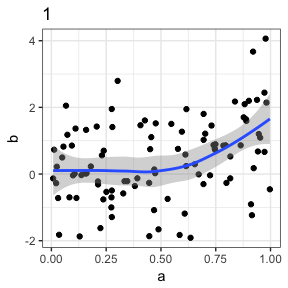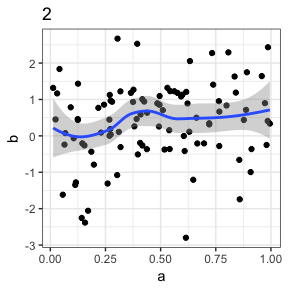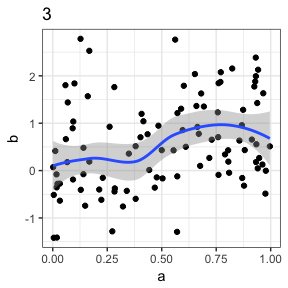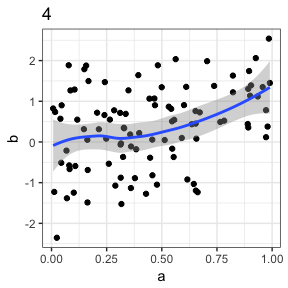#### dotplots

This function creates a dot plot for every group/unit in the data.

library(plotrr)
a <- runif(400, min = 0, max = 1)
b <- a + rnorm(400, mean = 0, sd = 1)
c <- rep(c(1:4), times = 100)
data <- data.frame(a, b, c)
dotplots("a", "b", "c", data, 20)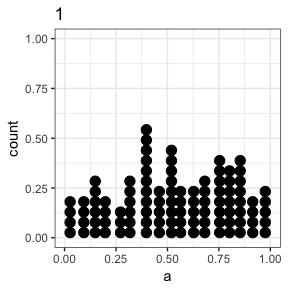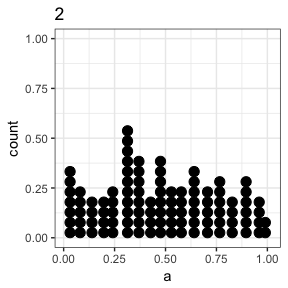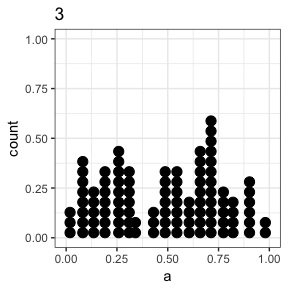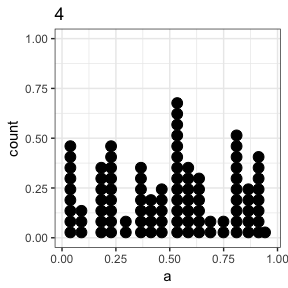#### violinplots

This function creates a violin plot for every group/unit in the data.

library(plotrr)
a <- runif(400, min = 0, max = 1)
b <- a + rnorm(400, mean = 0, sd = 1)
c <- rep(c(1:4), times = 100)
data <- data.frame(a, b, c)
violinplots("a", "b", "c", data)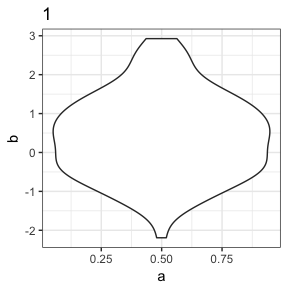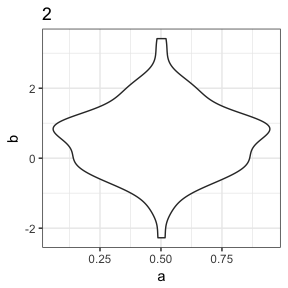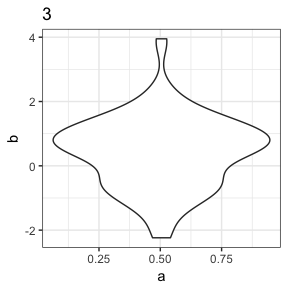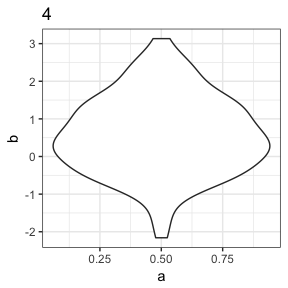### Functions that evaluate correlations between measures within groups/units

Another set of functions helps the researcher evaluate correlations between measures within groups/units. groupcor returns a tibble data frame with group/unit identifiers and the correlation coefficient between two measures for each group/unit in a data frame. groupcorplot performs a similar function and returns a figure that plots the correlation coefficient between measures within groups/units.

These functions can help researchers identify important patterns across groups/units. They can also help scholars check the correlation between competing measures - such as different measures of state human rights practices - within units. This is one way to check the construct validity of the measures (Trochim & Donnelly, 2008). Specifically, they can help scholars check the different criterion-related validities - predictive validity, concurrent validity, convergent validity, and discriminant validity. Finally, these functions also might be useful to generate new empirical puzzles; if measures disagree in important cases, why is this the case?

### Other visualization functions

In addition to these functions, the package includes histplots, which creates histograms of a measure for each group/unit. Here is an example.

library(plotrr)
a <- rnorm(400, mean = 2, sd = 100)
b <- a + rnorm(400, mean = 0, sd = 1)
c <- rep(c(1:4), times = 100)
data <- data.frame(a, b, c)
histplots("a", "b", "c", data, 5)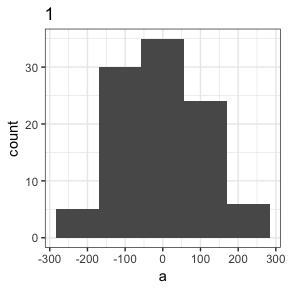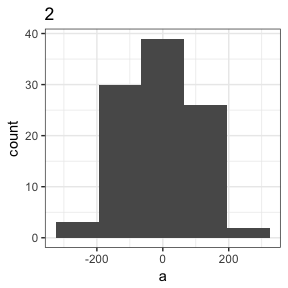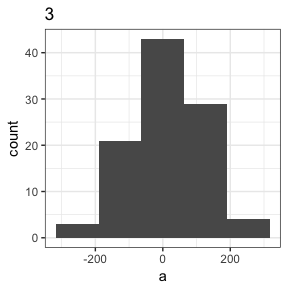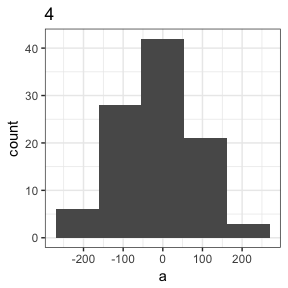It also includes bivarrugplot, which returns a plot of the bivariate relationship between two measures alongside a rugplot of each measure.

library(plotrr)
a <- runif(1000, min = 0, max = 1)
b <- a + rnorm(1000, mean = 0, sd =1)
data <- data.frame(a, b)
bivarrugplot("a", "b", data)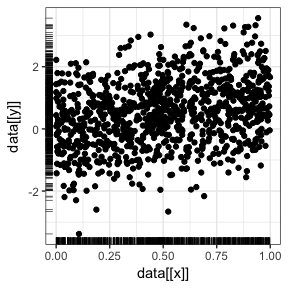### Other functions

Finally, the package also contains several “helper,” or convenience, functions. clear effectively clears the R terminal. lengthunique calculates the number of uniques values in a vector. makefacnum converts factor vectors numeric vectors.